### Home > CALC > Chapter 1 > Lesson 1.4.1 > Problem1-138

1-138.
1. Given a portion of the graph of f(x) below: 1-138 eTool (Desmos). Homework Help ✎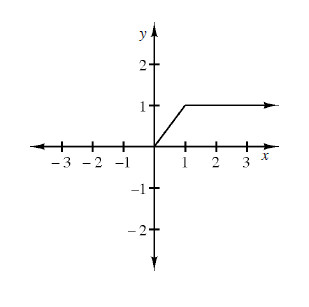1. Sketch the rest of f(x) if f(x) is even and find A(f, 3 ≤ x ≤ 3).

2. Sketch the rest of f(x) if f(x) is odd and find A(f, −3 ≤ x ≤ 3).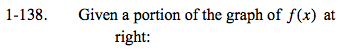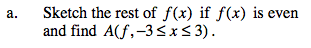If a function is even, it is symmetric about the y-axis.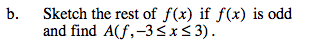If a function is odd, it also has 180º rotational symmetry about the origin.

Because of the symmetry of the areas, A(f, −3 ≤ x ≤ 3) = 0.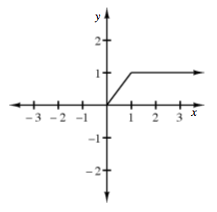Use the eTool below to visualize this problem.
Click the link at right for the full version of the eTool: Calc 1-138 HW eTool